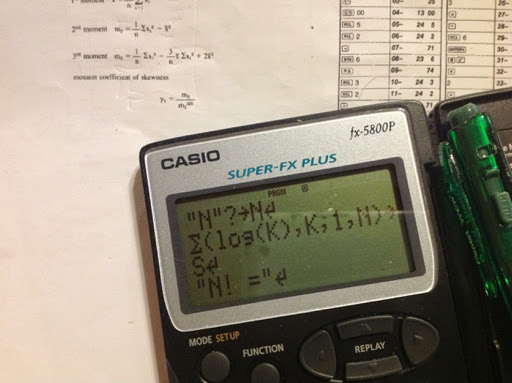## Friday, January 16, 2015

### fx-5800p: Large Factorials, Length of Daylight, & Moments/Skewnessfx-5800p: Large Factorials, Length of Daylight, & Moments/Skewness

Large Factorials

This allows the user to calculate n! for any positive n, even n > 69.

Program BIGFACT
"N"?→N
Σ(log(K),K,1,N) → S
"N = "
10^(Frac(S)) ◢
"* 10^( )"
Int(S)

Examples:
36! ≈ 3.719933268 * 10^ 41
134! ≈ 1.992942746 * 10^ 228

Length of Daylight

D = days after March 21. A 365 day year is assumed.

Program DAYLIGHT
"DAYS AFTER"
"MARCH 21"? → D
Deg
23.45 * sin(D*.9856) → T
"LATITUDE"
"D ● M ● S ●"? → L // for ● press [ ° ' " ]
cos⁻¹ (-tan(L) * tan(T) ) → A
2 * A / 15 → H
"HOURS="
H

Example:
D = 302 (January 17)
L = 40° N (+40°)
Result: H = 9.525548719 hours

D = 302 (January 17)
L = 40° S (-40°)
Result H = 14.47445128

Moments/Skewness

Source: HP 33 Math Pak, 1979

Formulas:

1st Moment: A = Σx ÷ n
2nd Moment: B = Σ(x^2) ÷ n - A^2
3rd Moment: C = (Σ(x^3) - 3 * A * Σ(x^2)) ÷ n + 2 * A^3
Coefficient of Skewness: D = C / B^1.5

Program MOMENTS
0 → N // Initialization
0 → U
0 → V
0 → W
Lbl 0 // Main Loop
N + 1 → N
U + X → U
V + X^2 → V
W + X^(3) → W
"NO. OF PTS."
N ◢
"ANOTHER PT.?" // are you done?
"0=NO 1=YES"
? → Y
Y ≠ 0 ⇒ Goto 0
U ÷ N → A // analysis
V ÷ N - A^2 → B
(W - 3*A*V) ÷ N + 2 * A^(3) → C
C ÷ B^(1.5) → D
"1ST MOMENT"
A ◢
"2ND MOMENT"
B ◢
"3RD MOMENT"
C ◢
"SKEWNESS"
D

Example:

Data: 1.5, 2.8, 2.9, 3.6, 4.7

Results:
1st Moment = 3.1
2nd Moment = 1.1
3rd Moment = 0.018
4th Moment = 0.0156021151

See you all next time - have a great weekend! Go have some fun!

This blog is property of Edward Shore. 2015